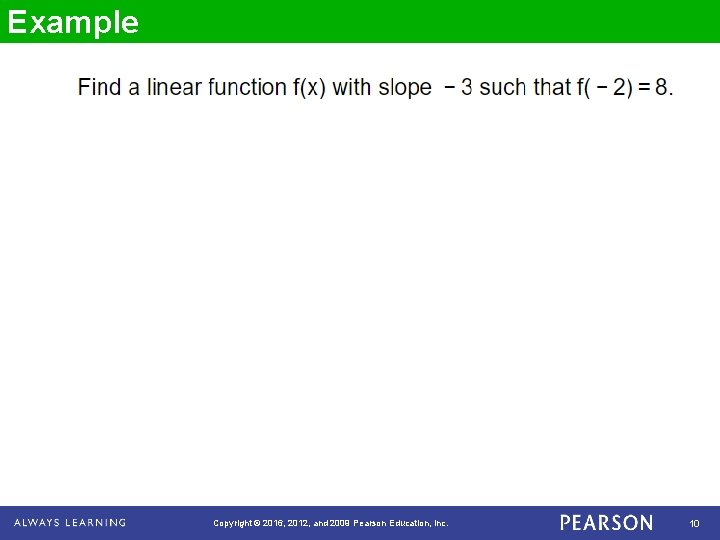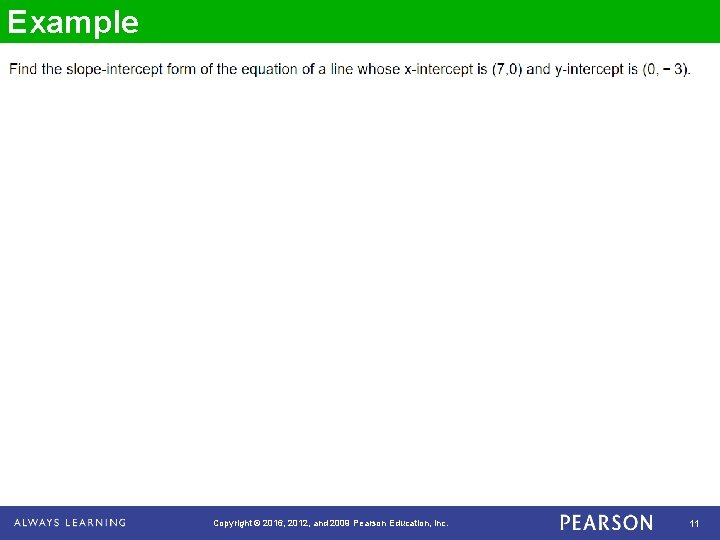# Chapter 3 Graphing Linear Equations Section 6 Equations

• Slides: 11Chapter 3 Graphing Linear Equations Section 6 Equations of Lines Copyright © 2016, 2012, and 2009 Pearson Education, Inc. 1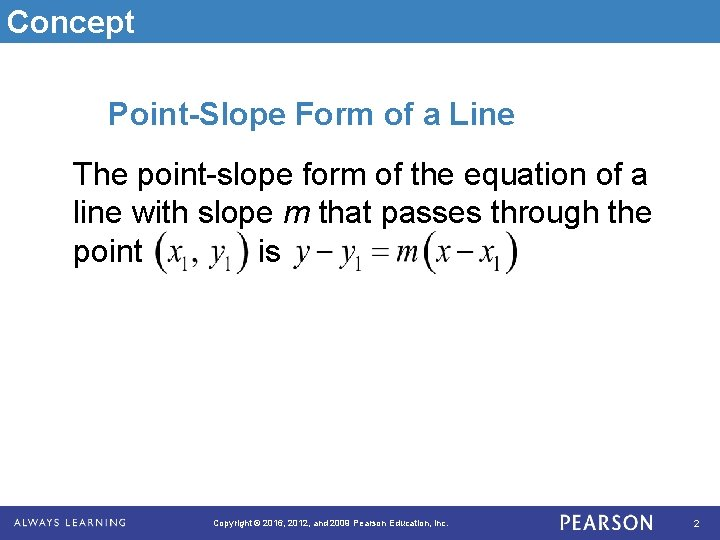Concept Point-Slope Form of a Line The point-slope form of the equation of a line with slope m that passes through the point is Copyright © 2016, 2012, and 2009 Pearson Education, Inc. 2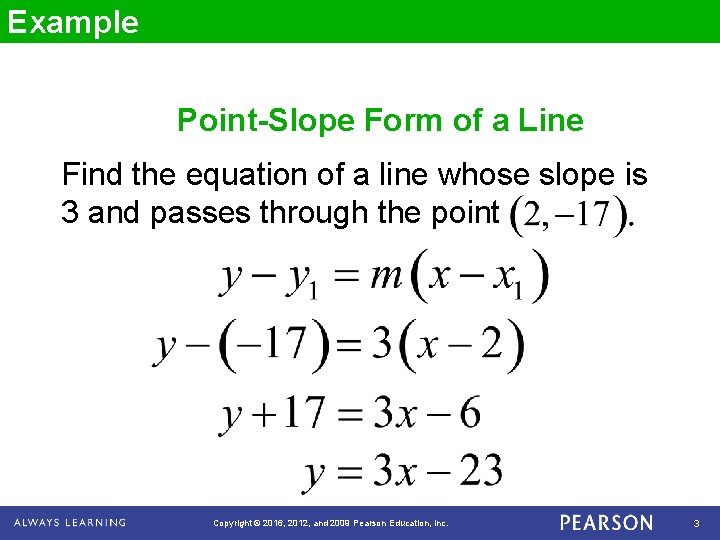Example Point-Slope Form of a Line Find the equation of a line whose slope is 3 and passes through the point Copyright © 2016, 2012, and 2009 Pearson Education, Inc. 3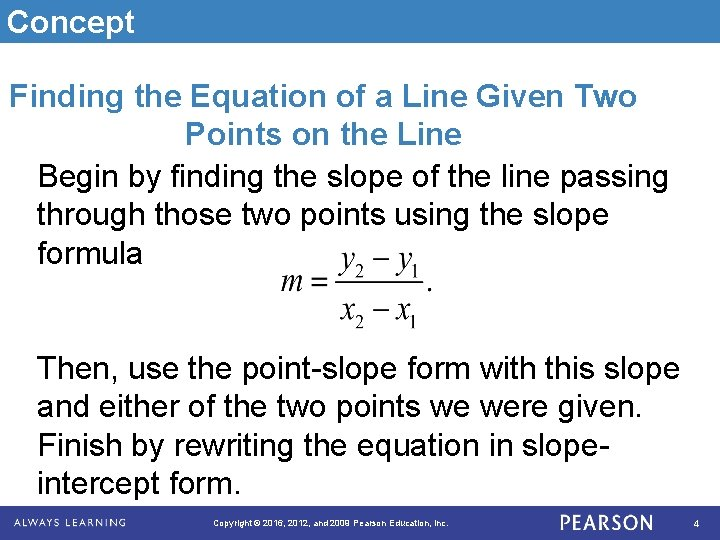Concept Finding the Equation of a Line Given Two Points on the Line Begin by finding the slope of the line passing through those two points using the slope formula Then, use the point-slope form with this slope and either of the two points we were given. Finish by rewriting the equation in slopeintercept form. Copyright © 2016, 2012, and 2009 Pearson Education, Inc. 4Example Finding the Equation of a Line Given Two Points on the Line Find the equation of a line that passes through the points Copyright © 2016, 2012, and 2009 Pearson Education, Inc. 5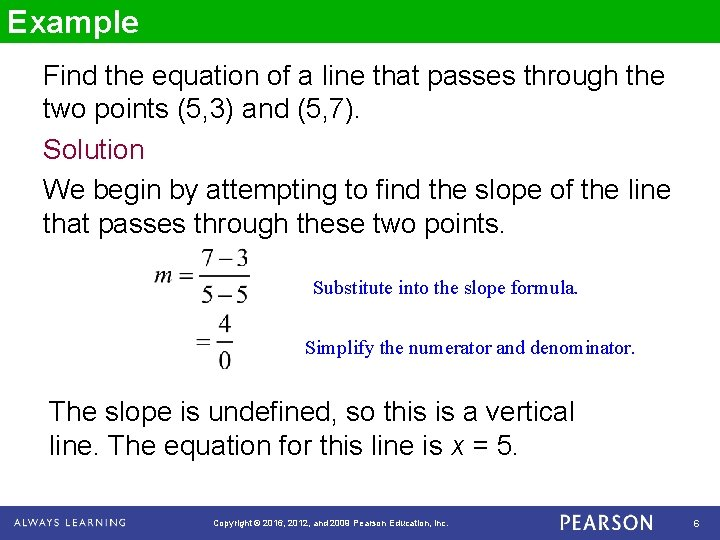Example Find the equation of a line that passes through the two points (5, 3) and (5, 7). Solution We begin by attempting to find the slope of the line that passes through these two points. Substitute into the slope formula. Simplify the numerator and denominator. The slope is undefined, so this is a vertical line. The equation for this line is x = 5. Copyright © 2016, 2012, and 2009 Pearson Education, Inc. 6Example In 2001, a marathon has 4, 500 registered entries. By 2004, the entries had dropped to 3000. Find a linear equation that describes the number (y) of entries x years after 2001. Since x represents the number of years after 2001, then x = 0 for the year 2001 and x = 3 for the year 2004. This tells us the two points on the line are (0, 4500) and (3, 3000). We begin by calculating the slope of the line. Copyright © 2016, 2012, and 2009 Pearson Education, Inc. 7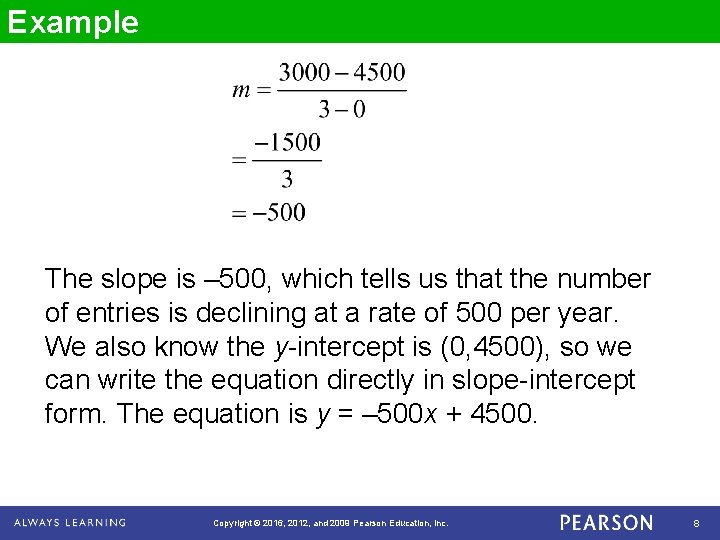Example The slope is – 500, which tells us that the number of entries is declining at a rate of 500 per year. We also know the y-intercept is (0, 4500), so we can write the equation directly in slope-intercept form. The equation is y = – 500 x + 4500. Copyright © 2016, 2012, and 2009 Pearson Education, Inc. 8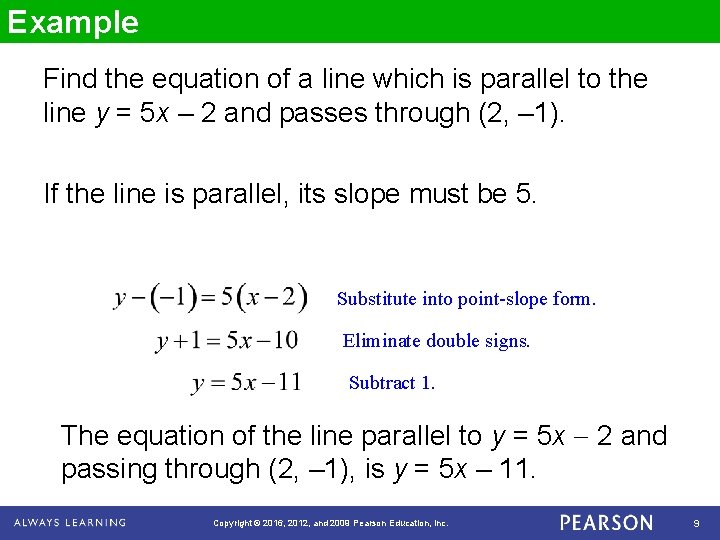Example Find the equation of a line which is parallel to the line y = 5 x – 2 and passes through (2, – 1). If the line is parallel, its slope must be 5. Substitute into point-slope form. Eliminate double signs. Subtract 1. The equation of the line parallel to y = 5 x 2 and passing through (2, – 1), is y = 5 x – 11. Copyright © 2016, 2012, and 2009 Pearson Education, Inc. 9